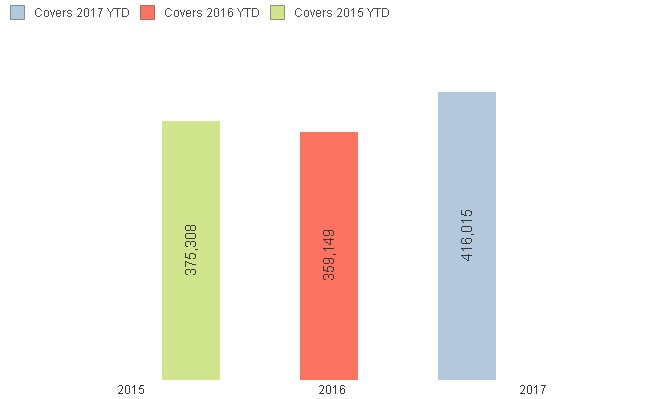# QlikView App Dev

Discussion Board for collaboration related to QlikView App Development.

Announcements
Support Case Portal has moved to Qlik Community! Read the FAQs to start exploring Support resources.
cancel
Showing results for
Did you mean:Contributor III

## Multiple Dates within Expression

Hi

Is it possible to create an expression that contains the equivalent of an OR statement

SQL would be;

select sum(sales)

Where (Yearfield = 2017 AND Datefield <= '2017/05/10')

OR (Yearfield = 2016 AND Datefield <= '2016/05/10')

OR (Yearfield = 2015 AND Datefield <= '2015/05/10')

So I would end up with numbers for YTD 3 year comparison.

I want to use this to calculate an average so I can see how the average plots year on year after the same period.

1 Solution

Accepted SolutionsContributor III
Author

I have been able to find the solution '+' is OR

For;

(Cover=1 AND Year = Current )

OR (Cover=1 AND Year = Previous AND date <= today last year)

OR (Cover=1 AND Year = 2 yrs ago AND date <= today 2 yrs ago)

Expression =;

=sum({<[Cover]={'1'},[Financial Year]={"\$(v.Date.Current.Year)"}>

+<[Cover]={'1'} ,[Financial Year]={"\$(v.Date.Year.Prior)"},[Transaction Date]={"<=\$(v.Date.Date.TodayLastYear)"}>

+<[Cover]={'1'} ,[Financial Year]={"\$(v.Date.Year.Prior2)"},[Transaction Date]={"<=\$(v.Date.Date.Today2YearsAgo)"}>

}[Quantity Sold])

4 RepliesAnonymous
Not applicable

you could try

...

where yeartodate(Datefield,0) or yeartodate(Datefield,-1) or yeartodate(Datefield,-2) ...

You could also do this in a loop in combination with concatenateSpecialist

Hi, John!

Would it be usefull?

=sum(if(Yearfield = 2017 AND Datefield <= '2017/05/10',sales,0))+

sum(if(Yearfield = 2016 AND Datefield <= '2016/05/10',sales,0))+

sum(if(Yearfield = 2015 AND Datefield <= '2015/05/10',sales,0))Contributor III
Author

Hi,

Thanks for your replies, unfortunately neither resolved my issue

I've tried another example below;

=sum({<[Cover]={'1'}

AND (

([Financial Year]={"\$(v.Date.Current.Year)"},[Transaction Date]={"<=\$(v.Date.Yesterday)"})

OR ([Financial Year]={"\$(v.Date.Year.Prior)"},[Transaction Date]={"<=\$(v.Date.Date.TodayLastYear)"})

OR ([Financial Year]={"\$(v.Date.Year.Prior2)"},[Transaction Date]={"<=\$(v.Date.Date.Today2YearsAgo)"})

)

>}[Quantity Sold])

This would produce a simple chart as in the example below however the example below has the 3 date ranges as separate expressions, I need all 3 as 1 expression as I need to over lay a line graph, which is currently resulting in 3 separate dots rather than a single trend line.Contributor III
Author

I have been able to find the solution '+' is OR

For;

(Cover=1 AND Year = Current )

OR (Cover=1 AND Year = Previous AND date <= today last year)

OR (Cover=1 AND Year = 2 yrs ago AND date <= today 2 yrs ago)

Expression =;

=sum({<[Cover]={'1'},[Financial Year]={"\$(v.Date.Current.Year)"}>

+<[Cover]={'1'} ,[Financial Year]={"\$(v.Date.Year.Prior)"},[Transaction Date]={"<=\$(v.Date.Date.TodayLastYear)"}>

+<[Cover]={'1'} ,[Financial Year]={"\$(v.Date.Year.Prior2)"},[Transaction Date]={"<=\$(v.Date.Date.Today2YearsAgo)"}>

}[Quantity Sold])Community Browser